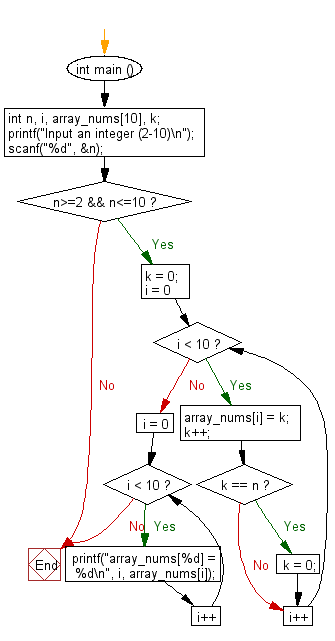﻿ C : Fill array elements with numbers 0 to n - 1 repeatedly

# C Exercises: Fill the array elements with number o to n – 1 repeated times

## C Basic Declarations and Expressions: Exercise-128 with Solution

Write a C program that reads an array of integers (length 10), fills it with numbers from o to a (given number) n – 1 repeatedly, where 2 ≤ n ≤ 10.

Sample Solution:

C Code:

``````#include <stdio.h>
int main ()
{
int n, i, array_nums, k;

// Prompt user for input
printf("Input an integer (2-10)\n");
scanf("%d", &n);

if (n >= 2 && n <= 10)
{
k = 0;

// Loop to fill array with sequential numbers, starting from 0
for (i = 0; i < 10; i++)
{
array_nums[i] = k;
k++;

if (k == n)
{
k = 0;
}
}

// Loop to print array elements
for (i = 0; i < 10; i++)
{
printf("array_nums[%d] = %d\n", i, array_nums[i]);
}
}
return 0; // End of program
}
``````

Sample Output:

```Input an integer (2-10)
8
array_nums = 0
array_nums = 1
array_nums = 2
array_nums = 3
array_nums = 4
array_nums = 5
array_nums = 6
array_nums = 7
array_nums = 0
array_nums = 1
```

Flowchart:C programming Code Editor:

What is the difficulty level of this exercise?

Test your Programming skills with w3resource's quiz.

﻿